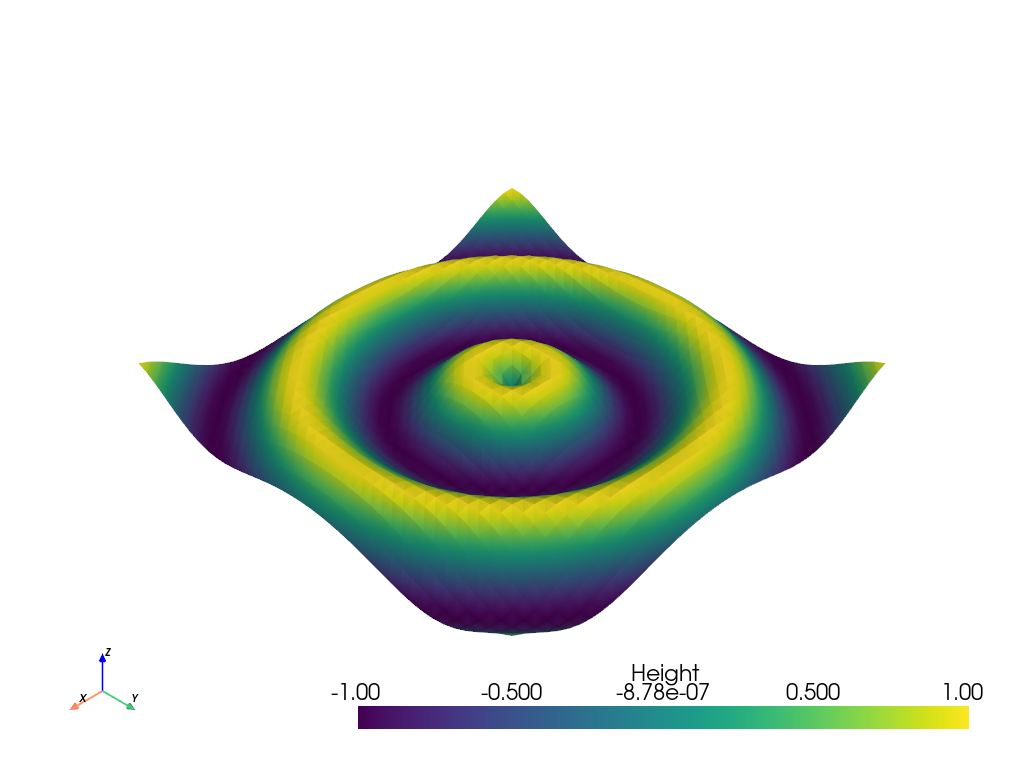# Create a GIF Movie¶

Generate a moving gif from an active plotterOut:

```Orient the view, then press "q" to close window and produce movie
```

```import pyvista as pv
import numpy as np

x = np.arange(-10, 10, 0.25)
y = np.arange(-10, 10, 0.25)
x, y = np.meshgrid(x, y)
r = np.sqrt(x ** 2 + y ** 2)
z = np.sin(r)

# Create and structured surface
grid = pv.StructuredGrid(x, y, z)

# Create a plotter object and set the scalars to the Z height
plotter = pv.Plotter()

print('Orient the view, then press "q" to close window and produce movie')

# setup camera and close
plotter.show(auto_close=False)

# Open a gif
plotter.open_gif("wave.gif")

pts = grid.points.copy()

# Update Z and write a frame for each updated position
nframe = 15
for phase in np.linspace(0, 2 * np.pi, nframe + 1)[:nframe]:
z = np.sin(r + phase)
pts[:, -1] = z.ravel()
plotter.update_coordinates(pts)
plotter.update_scalars(z.ravel())
plotter.write_frame()

# Close movie and delete object
plotter.close()
```

Total running time of the script: ( 0 minutes 8.089 seconds)

Gallery generated by Sphinx-Gallery